# Make Genome-level Trellis Graph

Author: Zuguang Gu ( z.gu@dkfz.de )

Date: 2017-11-01

Package version: 1.11.1

Trellis graph is a type of graph which splits data by certain conditions and visualizes subset of data in each category parallel. In genomic data analysis, the conditional variable is mostly the chromosome. The advantage of Trellis graph is it can easily reveal multiple variable relationship behind the data. In R, lattice and ggplot2 package can make Trellis graph, however, specially for whole genome level plot, they are limited in:

• Chromosomes have different length. But in lattice/ggplot2, panel width for each chromosome are the same, so short chromosomes will be extended with empty areas which sometimes is a waste of space. (just find out latticeExtra allows different panel width.)
• Genomic data are more multiple dimensional, which means, there are always more than one tracks for chromosomes that to be compared. Unfortunately, lattice/ggplot2 can only plot one track at one time.

For a single continuous region, multiple tracks are supported in ggbio and Gviz. But if you want to compare more than one regions, things will be complex. Due to the design of ggbio or Gviz, it will not be so efficient to visualize e.g. larger than 10 regions at a same time.

Here, gtrellis provides a flexible way to arrange genomic categories. It has following advantages:

• The layout is flexible that it is good at visualizing many genomic categories at a same time (e.g. 24 human chromosomes).
• Genomic category is not restricted in chromosome, it can be any type (e.g. genes).
• Support multiple tracks.
• Graphics in tracks can be highly customized.

## Basic design

gtrellis aims to arrange genomic categories as Trellis style and supports multiple tracks for visualization. In this package, initializating the layout and adding graphics are independent. After initialization of the layout, intersection between tracks and genomic categories are named cell or panel, and each cell is an independent plotting region (actually, each cell is a viewport in grid system) that self-defined graphics can be added afterwards.

gtrellis is implemented in grid graphic system, so, in order to add graphics in each cell, users need to use low-level graphic functions (`grid.points`, `grid.lines`, `grid.rect`, …) which are quite similar as those in classic graphic system.

## Initialize the layout

`gtrellis_layout()` is used to create the global layout. By default, it initializes the layout with hg19 and puts all chromosomes in one row. Each chromosome has only one track and range on y-axis is 0 to 1.

``````library(gtrellis)
gtrellis_layout()
``````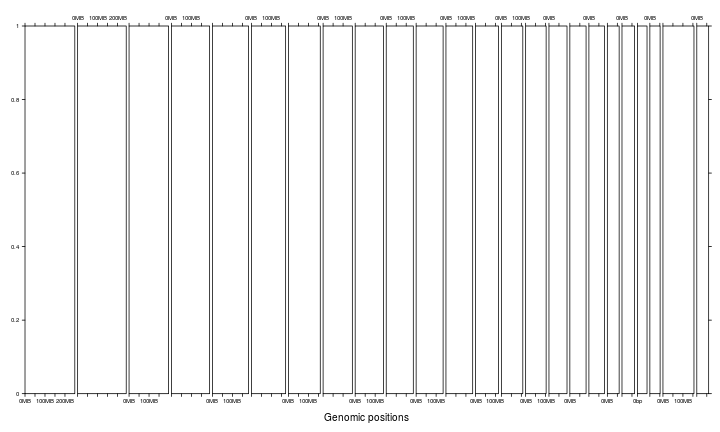`category` can be used to set subset of chromosomes as well as the order of chromosomes. `gtrellis_show_index()` here is an assistant function to add the information to each cell, just for demonstration purpose in this vignette.

``````gtrellis_layout(category = c("chr3", "chr1"))
gtrellis_show_index()
``````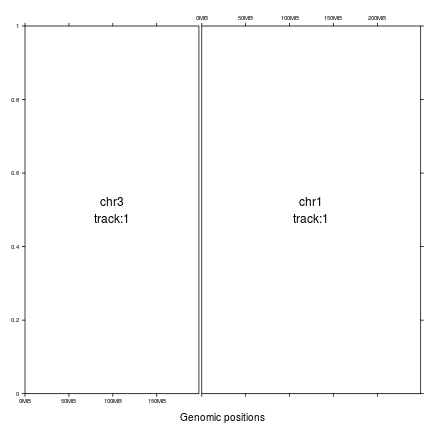Other species are also supported as long as corresponding chromInfo files exist on UCSC ftp. E.g. chromInfo file for mouse (mm10) is http://hgdownload.cse.ucsc.edu/goldenpath/mm10/database/chromInfo.txt.gz. Since there may be many short scaffolds in chromInfo file, if `category` is not specified, `gtrellis` will first remove these short scaffolds before making the plot. Also non-normal chromosomes (e.g. “chr1_xxxxxx”) will also be removed. Sometimes this detection is not always correct, if you find chromosomes shown on the plot is not what you expect, set `category` manually.

``````gtrellis_layout(species = "mm10")
gtrellis_show_index()
``````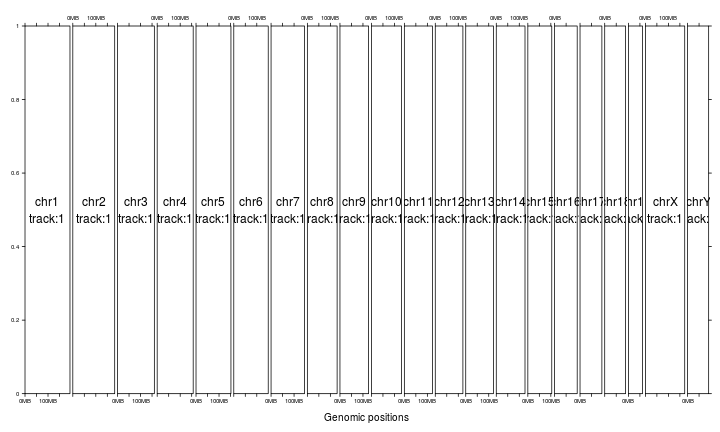You can put chromosomes on multiple rows by specifying `nrow` or/and `ncol`. For chromosomes in the same column, the corresponding width is the width of the longest chromosome in that column and short chromosomes will be extended with empty areas.

``````gtrellis_layout(nrow = 3)
gtrellis_show_index()
``````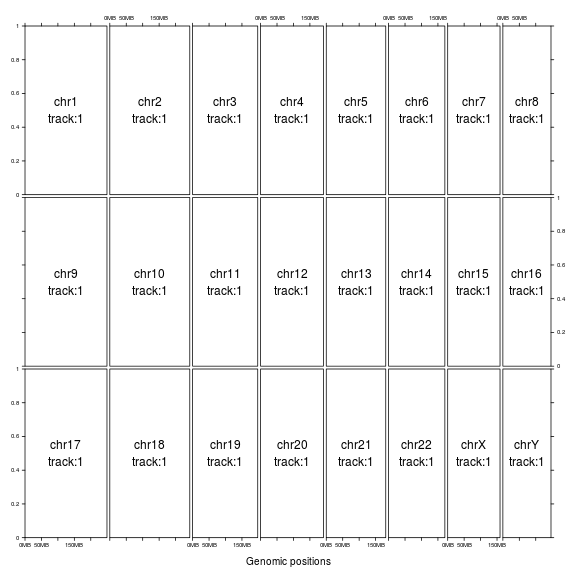``````gtrellis_layout(ncol = 5)
gtrellis_show_index()
``````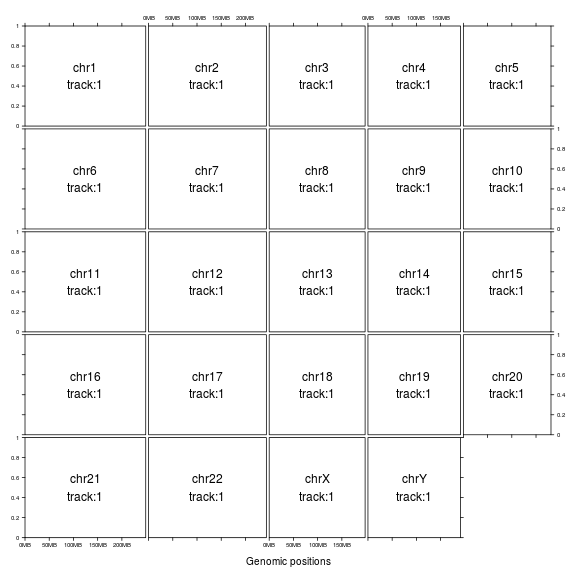You can set `byrow` argument to arrange chromosomes either by rows or by columns. As explained before, by default chromosomes in the same column will share the length of the longest one. It is better to put chromosomes with similar length in a same column.

``````gtrellis_layout(ncol = 5, byrow = FALSE)
gtrellis_show_index()
``````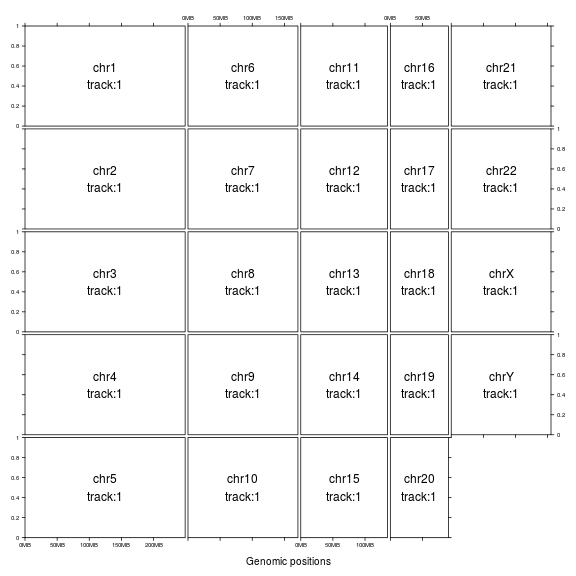If `equal_width` is set to `TRUE`, the layout will be a 'standard' Trellis layout. All chromosomes will share the same range on x-axis (length of the longest chromosome) and short chromosomes will be extended with empty areas.

``````gtrellis_layout(equal_width = TRUE)
gtrellis_show_index()
``````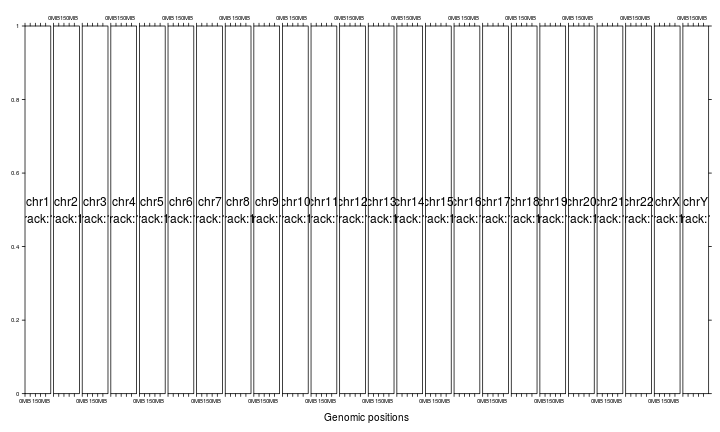Make all columns having equal width and also set multiple rows.

``````gtrellis_layout(ncol = 5, byrow = FALSE, equal_width = TRUE)
gtrellis_show_index()
``````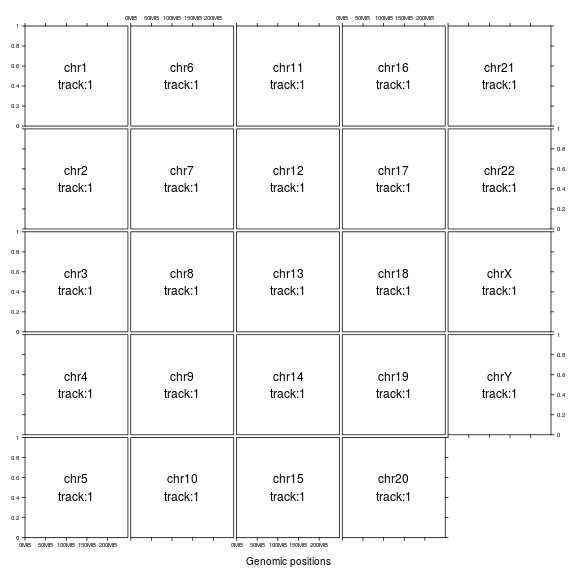There is also a 'compact' mode of the layout that when there are multiple rows, chromosomes on a same row can be put compactly without being aligned to corresponding columns. This mode saves a lot of white space but the drawback is that it is not easy to directly compare positions among chromosomes.

``````gtrellis_layout(nrow = 3, compact = TRUE)
gtrellis_show_index()
``````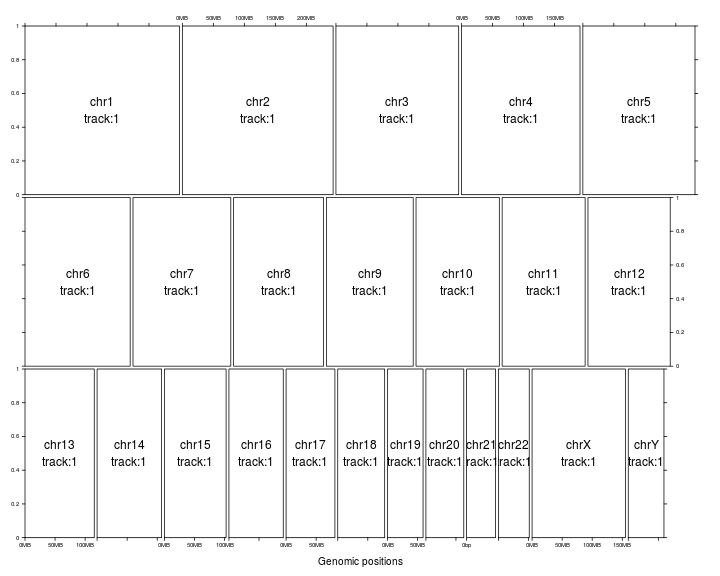Set gaps between chromosomes. Note if it is set as a numeric value, it should only be 0 (no gap).

``````gtrellis_layout(gap = 0)
``````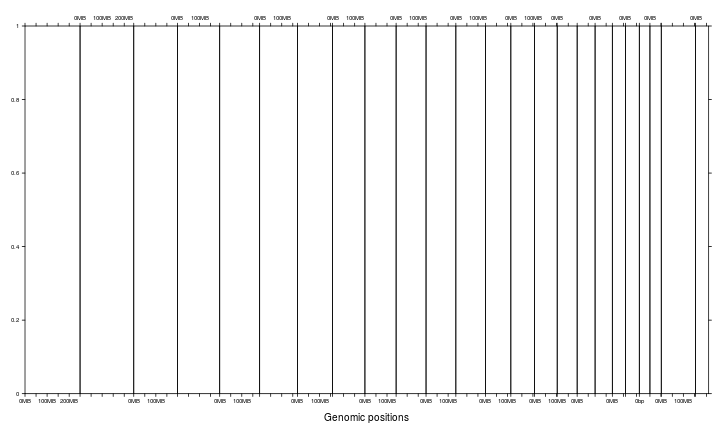Or `gap` can be a `unit` object.

``````gtrellis_layout(gap = unit(5, "mm"))
``````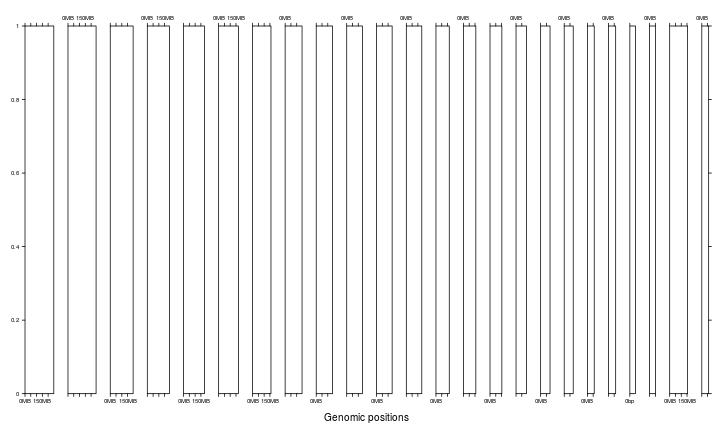When you arrange the layout with multiple rows, you can also set `gap` as length of two. In this case, the first element corresponds to the gaps between rows and the second corresponds to the gaps between columns.

``````gtrellis_layout(ncol = 5, gap = unit(c(5, 2), "mm"))
``````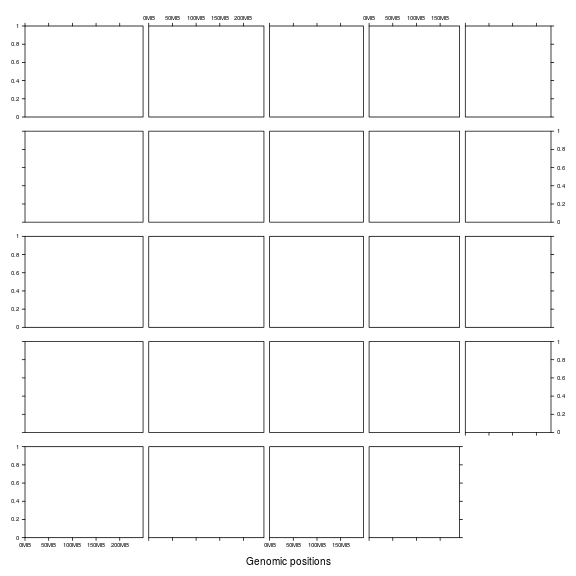There may be multiple tracks for chromosomes which describe multiple dimensional data. The tracks can be created by `n_track` argument.

``````gtrellis_layout(n_track = 3)
gtrellis_show_index()
``````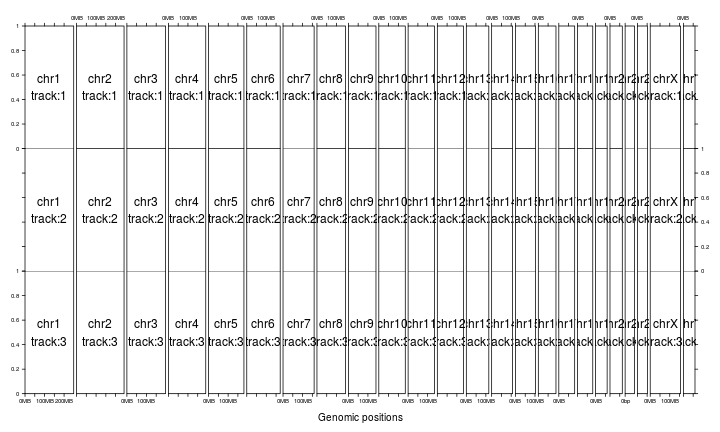By default, tracks share the same height. The height can be customized by `track_height` argument. If it is set as numeric values, it will be normalized as percent to the sum.

``````gtrellis_layout(n_track = 3, track_height = c(1, 2, 3))
``````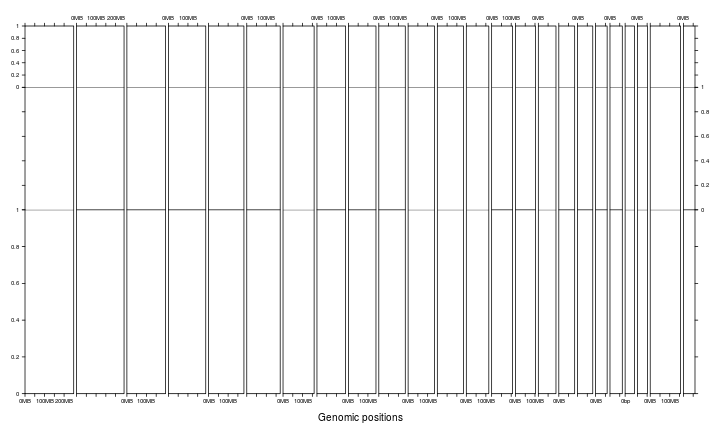`track_height` can also be a `unit` object.

``````gtrellis_layout(n_track = 3,
track_height = unit.c(unit(1, "cm"), unit(1, "null"), grobHeight(textGrob("chr1"))))
``````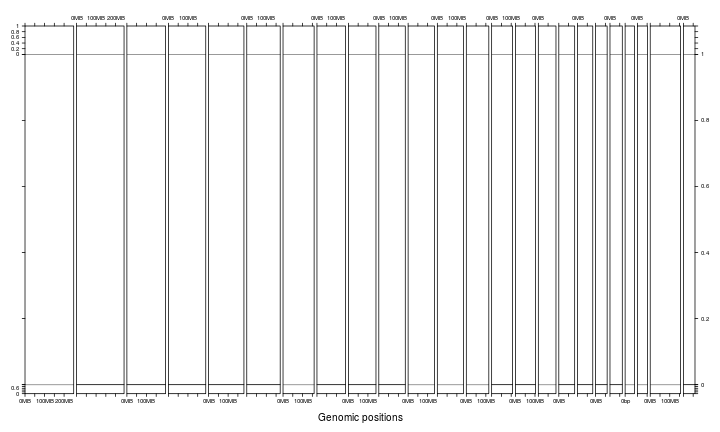`track_axis` controls whether to show y-axes. If certain value is set to `FALSE`, y-axis on corresponding track will not be drawn.

``````gtrellis_layout(n_track = 3, track_axis = c(FALSE, TRUE, FALSE), xaxis = FALSE, xlab = "")
``````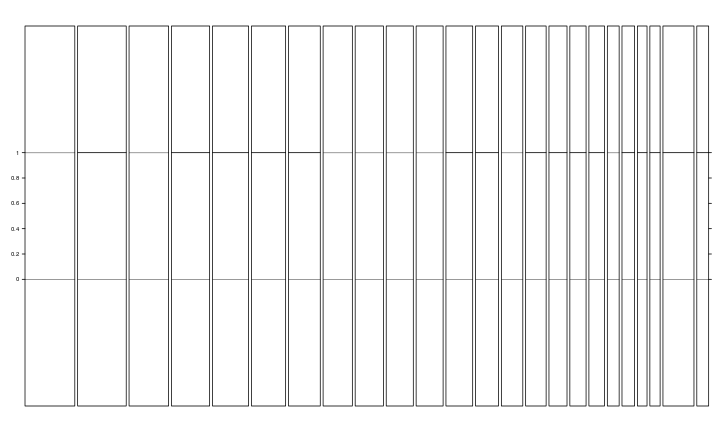Set y-lim by `track_ylim`. It should be a two-column matrix. But to make things easy, it can also be a vector and it will be filled into a two-column matrix by rows. If it is a vector with length 2, it means all tracks share the same y-lim.

``````gtrellis_layout(n_track = 3, track_ylim = c(0, 3, -4, 4, 0, 1000000))
``````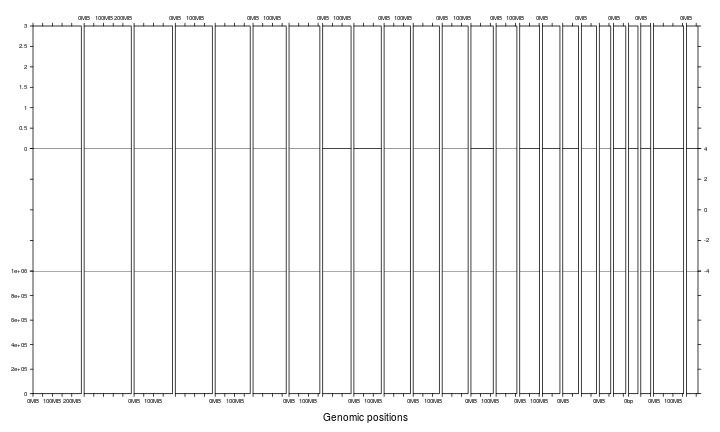Axis ticks are added on one side of rows or columns, `asist_ticks` controls whether to add axis ticks on the other sides. (You can compare following figure to the above one.)

``````gtrellis_layout(n_track = 3, track_ylim = c(0, 3, -4, 4, 0, 1000000), asist_ticks = FALSE)
``````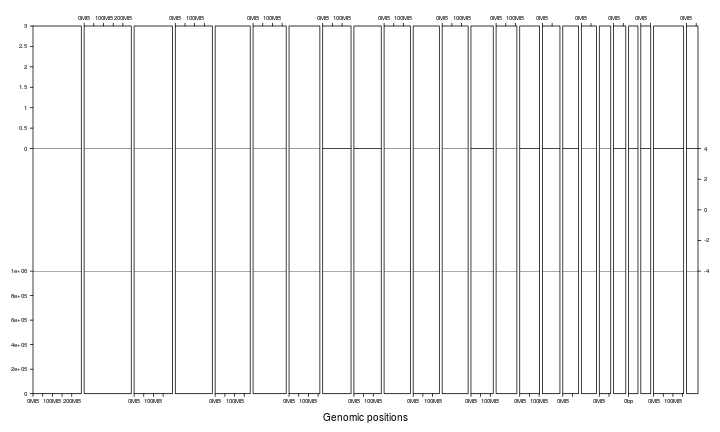Set x-label by `xlab` and set y-labels by `track_ylab`.

``````gtrellis_layout(n_track = 3, title = "title", track_ylab = c("", "bbbbbb", "ccccccc"), xlab = "xlab")
``````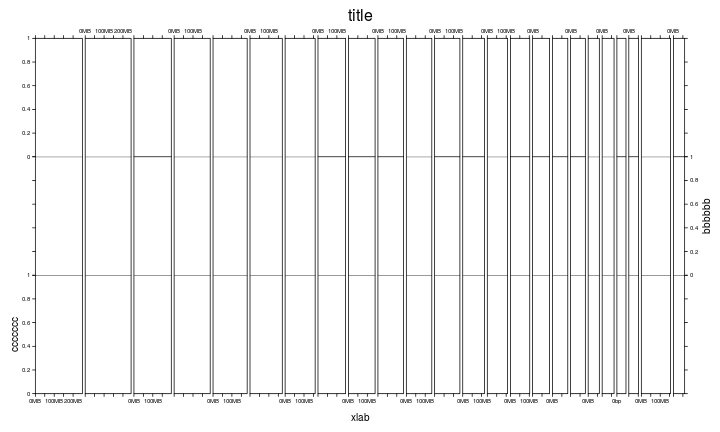Since chromosomes can have more than one tracks, following shows a layout with multiple columns and multiple tracks.

``````gtrellis_layout(n_track = 3, ncol = 4)
gtrellis_show_index()
``````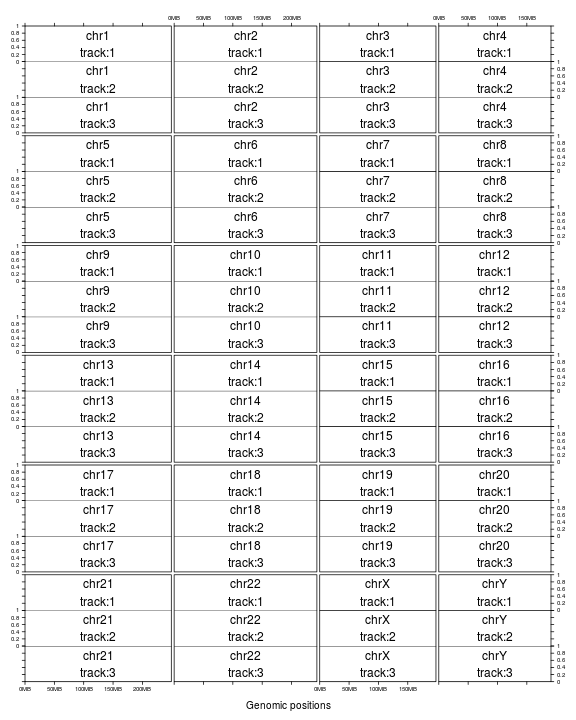Set `border` to `FALSE` to remove borders.

``````gtrellis_layout(n_track = 3, ncol = 4, border = FALSE, xaxis = FALSE, track_axis = FALSE, xlab = "")
gtrellis_show_index()
``````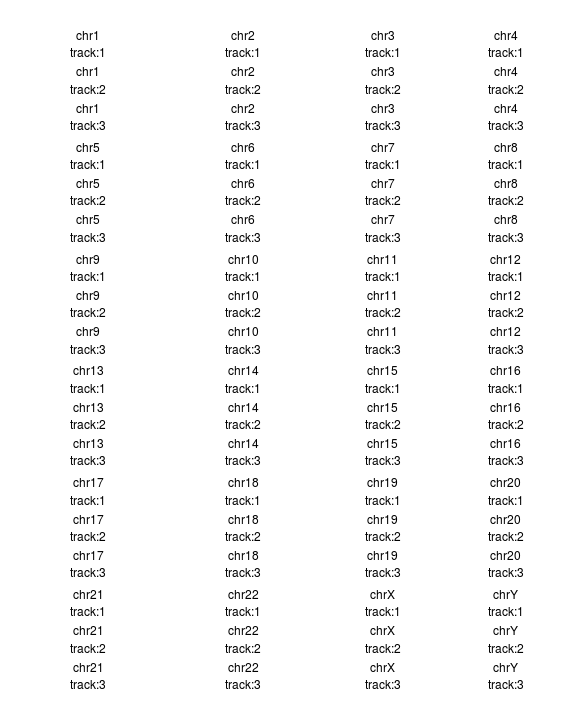After the initialization of the layout, each cell can be thought as an ordinary coordinate system. Then graphics can be added in afterwards.

### Pre-defined track

First we will introduce functions which add fixed types of graphics.

`add_points_track()` directly adds points at the middle points of corresponding genomic regions. The genomic region variable can be either a data frame or a `GRanges` object.

``````library(circlize)
bed = generateRandomBed()
gtrellis_layout(track_ylim = range(bed[]), nrow = 3, byrow = FALSE)
add_points_track(bed, bed[], gp = gpar(col = ifelse(bed[] > 0, "red", "green")))
``````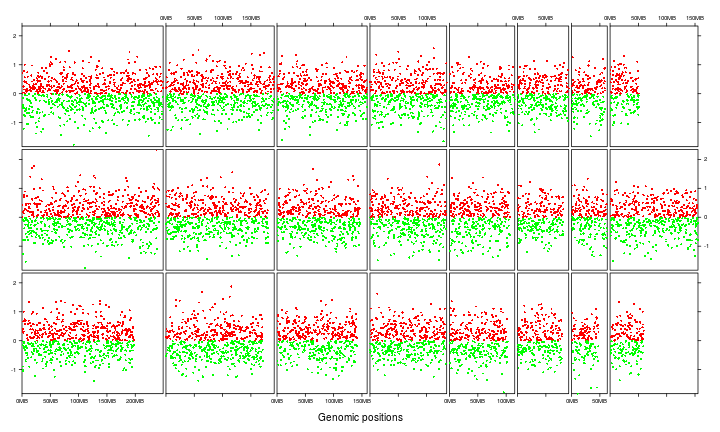`add_segments_track()` adds segments for corresponding regions.

``````bed = generateRandomBed(nr = 100)
gtrellis_layout(track_ylim = range(bed[]), nrow = 3, byrow = FALSE)
add_segments_track(bed, bed[], gp = gpar(col = ifelse(bed[] > 0, "red", "green"), lwd = 4))
``````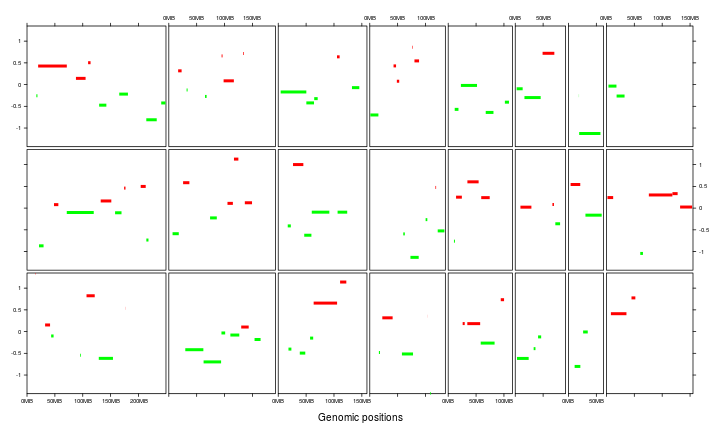`add_lines_track()` adds lines. Also it can draw areas below the lines (or above, depending on `baseline`).

``````bed = generateRandomBed(200)
gtrellis_layout(n_track = 2, track_ylim = rep(range(bed[]), 2), nrow = 3, byrow = FALSE)
add_lines_track(bed, bed[], area = TRUE, gp = gpar(fill = "grey", col = NA))
``````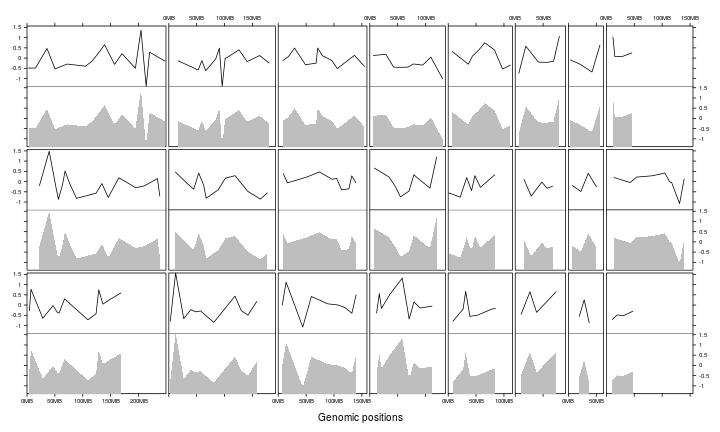`add_rect_track()` adds rectangles which is useful to draw bars.

``````col_fun = colorRamp2(c(-1, 0, 1), c("green", "black", "red"))
gtrellis_layout(track_ylim = range(bed[]), nrow = 3, byrow = FALSE)
add_rect_track(bed, h1 = bed[], h2 = 0,
gp = gpar(col = NA, fill = col_fun(bed[])))
``````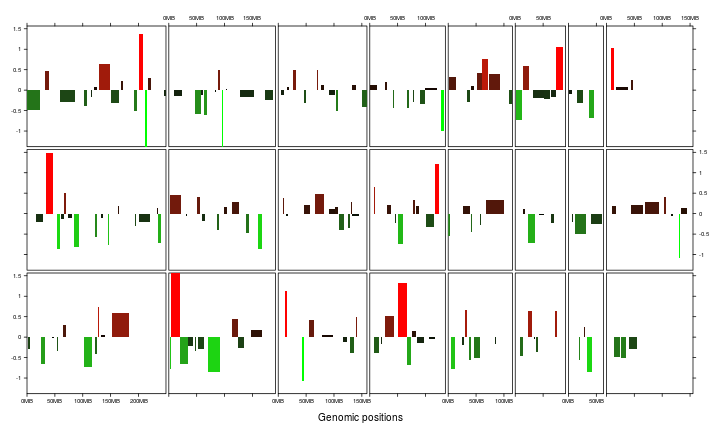`add_heatmap_track()` adds heatmap. Heatmap will fill the whole track vertically.

``````gtrellis_layout(nrow = 3, byrow = FALSE, track_axis = FALSE)
mat = matrix(rnorm(nrow(bed)*4), ncol = 4)
``````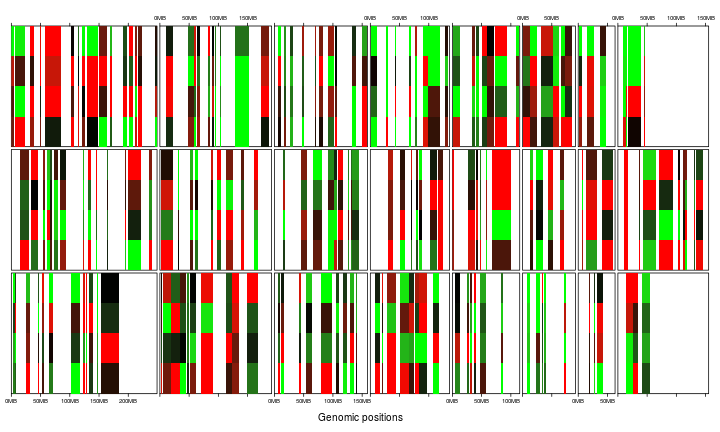By default, these pre-defined graphic functions draw in the next track. However, different types of graphics can be drawn in a same track by manually setting `track`.

``````col_fun = colorRamp2(c(-1, 0, 1), c("green", "black", "red"))
gtrellis_layout(track_ylim = range(bed[]), nrow = 3, byrow = FALSE)
add_rect_track(bed, h1 = bed[], h2 = 0, gp = gpar(col = NA, fill = col_fun(bed[])))
add_points_track(bed, bed[], track = current_track(), size = unit(abs(bed[])*5, "mm"))
``````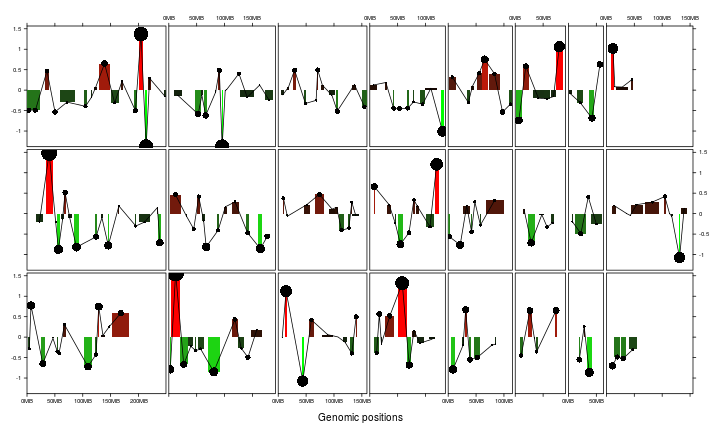### Customized track

More generally, `add_track()` allows adding self-defined graphics. Actually this is how `add_points_track()`, `add_segments_track()`, `add_lines_track()`, `add_rect_track()` and `add_heatmap_track()` are implemented.

The self-defined graphics are added by `panel_fun` argument which should be a function. `panel_fun` is applied to every genomic categories (e.g. chromosomes) and the input value of `panel_fun` is a subset of data which corresponds to the current chromosome. Following example simply shows how to add points by `panel_fun`.

``````bed = generateRandomBed()
gtrellis_layout(track_ylim = range(bed[]))
# `bed` inside `panel_fun` is a subset of the main `bed`
x = (bed[] + bed[]) / 2
y = bed[]
grid.points(x, y, pch = 16, size = unit(1, "mm"))
})
``````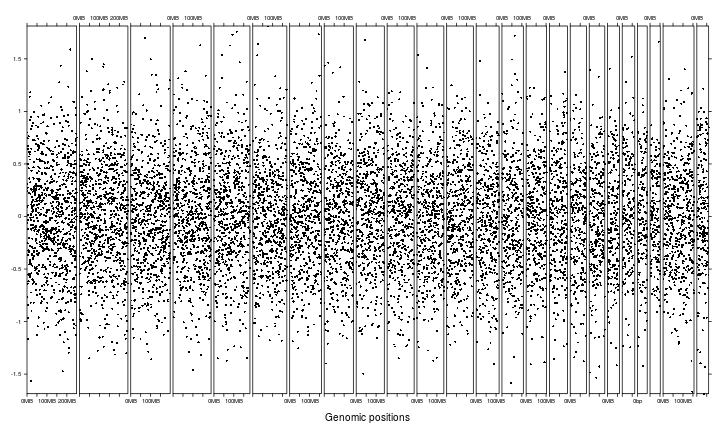If the input data is a `GRanges` object, the input variable in `panel_fun` is also a `GRanges` object.

``````gr = GRanges(seqnames = bed[],
ranges = IRanges(start = bed[],
end = bed[]),
score = bed[])
gtrellis_layout(track_ylim = range(gr\$score))
x = (start(gr) + end(gr)) / 2
y = gr\$score
grid.points(x, y, pch = 16, size = unit(1, "mm"))
})
``````Initialization and adding graphics are actually independent. Following example uses same code to add graphics but with different layout.

``````gtrellis_layout(nrow = 5, byrow = FALSE, track_ylim = range(bed[]))
x = (bed[] + bed[]) / 2
y = bed[]
grid.points(x, y, pch = 16, size = unit(1, "mm"))
})
``````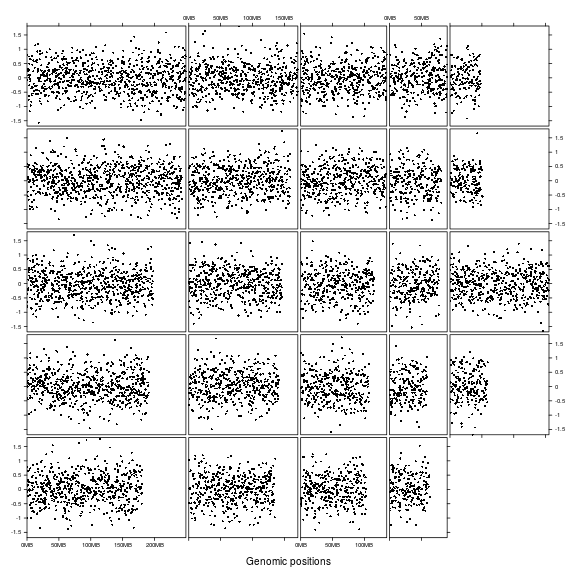## Examples

In following, we make rainfall plot as well as the density distribution of genomic regions (in the example below, `DMR_hyper` contains differentially methylated regions that show high methylation compared to control samples and in `DMR_hypo` the methylation is lower than control samples). Also, we manually add a track which contains chromosome names and a track which contains ideograms.

Density for genomic regions is defined as the percent of a genomic window that is covered by the input genomic regions.

``````load(system.file("extdata", "DMR.RData", package = "circlize"))
DMR_hyper_density = circlize::genomicDensity(DMR_hyper, window.size = 1e7)
``````
``````##    chr    start      end       pct
## 1 chr1        1 10000000 0.0038096
## 2 chr1  5000001 15000000 0.0019618
## 3 chr1 10000001 20000000 0.0029903
## 4 chr1 15000001 25000000 0.0024798
## 5 chr1 20000001 30000000 0.0020628
## 6 chr1 25000001 35000000 0.0024249
``````

Initialize the layout and add following four tracks:

1. chromosome names, simple text.
2. rainfall plot, first apply rainfall transformation and then add points.
3. genomic density, lines with area (actually it is polygons).
4. ideogram, rectangles.
``````gtrellis_layout(n_track = 4, ncol = 4, byrow = FALSE,
track_axis = c(FALSE, TRUE, TRUE, FALSE),
track_height = unit.c(2*grobHeight(textGrob("chr1")),
unit(1, "null"),
unit(0.5, "null"),
unit(3, "mm")),
track_ylim = c(0, 1, 0, 8, c(0, max(DMR_hyper_density[])), 0, 1),
track_ylab = c("", "log10(inter_dist)", "density", ""))

# track for chromosome names
# the use of `get_cell_meta_data()` will be introduced later
chr = get_cell_meta_data("name")
grid.rect(gp = gpar(fill = "#EEEEEE"))
grid.text(chr)
})

# track for rainfall plots
DMR_hyper_rainfall = circlize::rainfallTransform(DMR_hyper)
pch = 16, size = unit(1, "mm"), gp = gpar(col = "red"))

# track for genomic density
gp = gpar(fill = "pink"))

# track for ideogram
cytoband_chr = gr
grid.rect(cytoband_chr[], unit(0, "npc"),
width = cytoband_chr[] - cytoband_chr[], height = unit(1, "npc"),
default.units = "native", hjust = 0, vjust = 0,
gp = gpar(fill = circlize::cytoband.col(cytoband_chr[])))
grid.rect(min(cytoband_chr[]), unit(0, "npc"),
width = max(cytoband_chr[]) - min(cytoband_chr[]), height = unit(1, "npc"),
default.units = "native", hjust = 0, vjust = 0,
gp = gpar(fill = "transparent"))
})
``````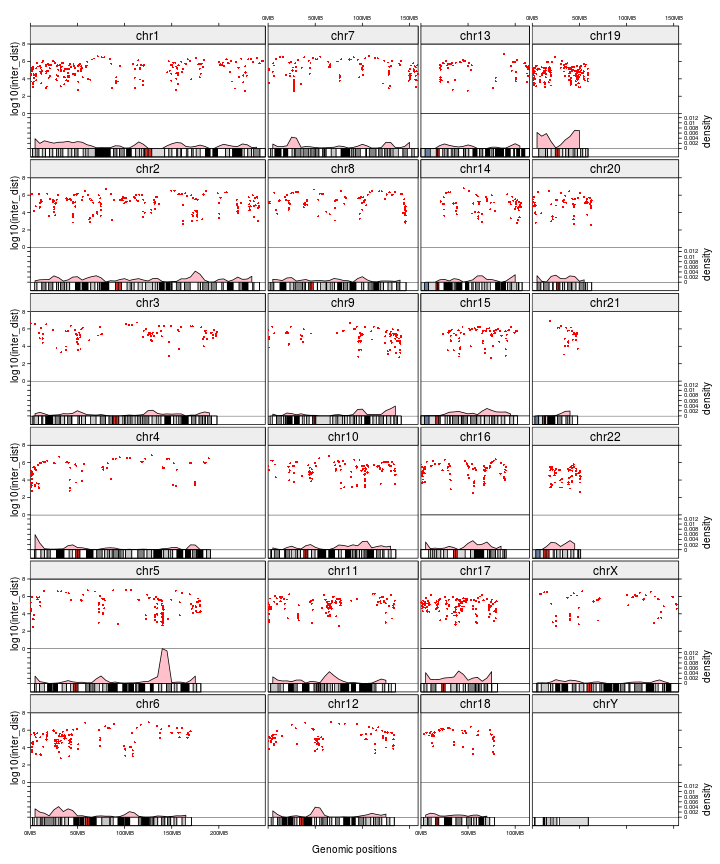Actually, you don't need to add name track and ideogram track manually. Name track and ideogram track can be added by `add_name_track` and `add_ideogram_track` arguments. Name track will be inserted before the first track and ideogram track will be inserted after the last track. So in following example, although we only specified `n_track` to 2, but the name track and ideogram track are also added, thus, the final number of track is 4.

In following example, we additionally add graphics for hypo-DMR as well so that direct comparison between different methylation patterns can be performed. Since rainfall plots for both hyper-DMR and hypo-DMR are added in a same track, we explicitly specify value of `track` argument to `current_track()` in `add_track()`.

``````DMR_hypo_density = circlize::genomicDensity(DMR_hypo, window.size = 1e7)
DMR_hypo_rainfall = circlize::rainfallTransform(DMR_hypo)

gtrellis_layout(n_track = 2, ncol = 4, byrow = FALSE,
track_axis = TRUE,
track_height = unit.c(unit(1, "null"),
unit(0.5, "null")),
track_ylim = c(0, 8, c(0, max(c(DMR_hyper_density[], DMR_hypo_density[])))),
track_ylab = c("log10(inter_dist)", "density"),

# put into a function and we will use it later
pch = 16, size = unit(1, "mm"), gp = gpar(col = "#FF000080"))
pch = 16, size = unit(1, "mm"), gp = gpar(col = "#0000FF80"))

# track for genomic density
gp = gpar(fill = "#FF000080"))
add_lines_track(DMR_hypo_density, DMR_hypo_density[], area = TRUE, track = current_track(),
gp = gpar(fill = "#0000FF80"))
}

``````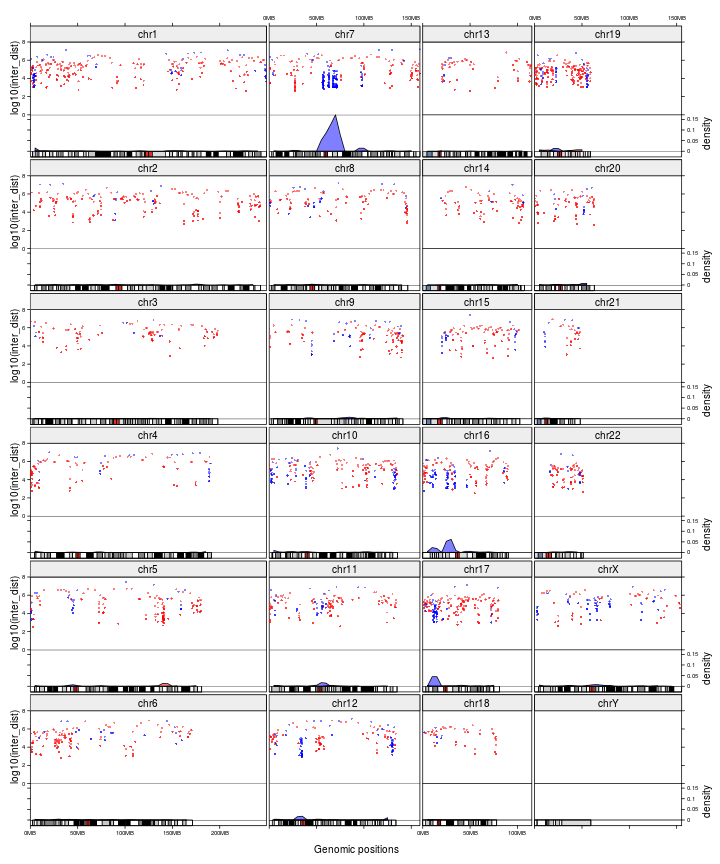Next we change the layout to the 'compact' mode without changing the code that adds graphics.

``````gtrellis_layout(n_track = 2, nrow = 4, compact = TRUE,
track_axis = TRUE,
track_height = unit.c(unit(1, "null"),
unit(0.5, "null")),
track_ylim = c(0, 8, c(0, max(c(DMR_hyper_density[], DMR_hypo_density[])))),
track_ylab = c("log10(inter_dist)", "density"),
``````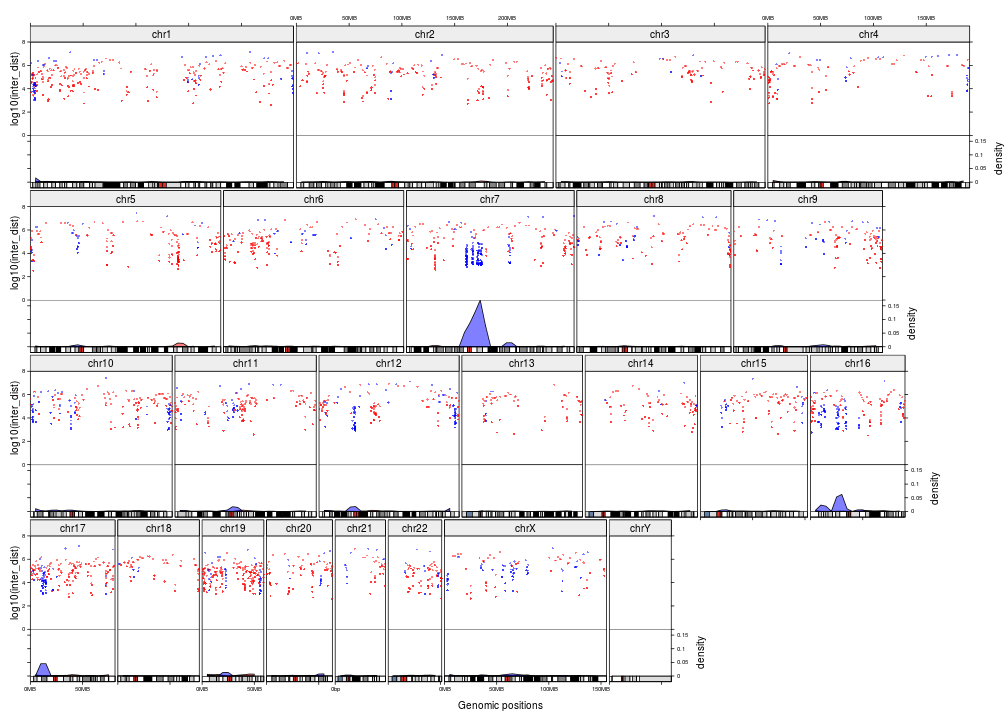By default, tracks are added from the first track to the last one. You can also add graphics in any specified chromosomes and tracks by specifying `category` and `track`.

``````all_chr = paste0("chr", 1:22)
letter = strsplit("MERRY CHRISTMAS!", "")[]
gtrellis_layout(nrow = 5)
for(i in seq_along(letter)) {
add_track(category = all_chr[i], track = 1, panel_fun = function(gr) {
grid.text(letter[i], gp = gpar(fontsize = 30))
})
}
``````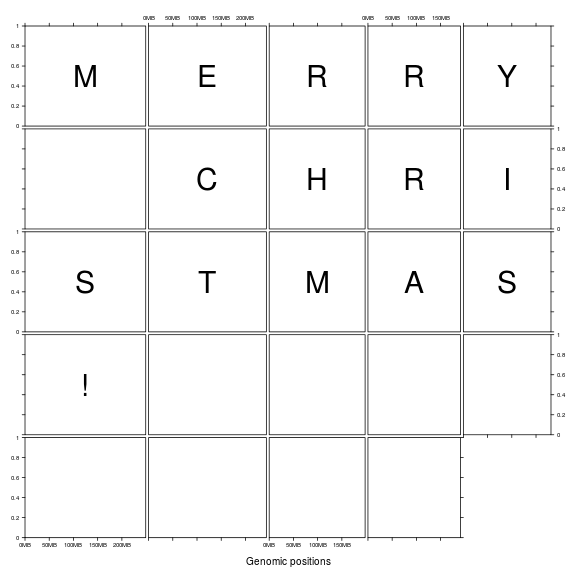Following code plots coverage for a tumor sample, its companion normal sample and the ratio of coverage. First prepare the data:

``````tumor_df = readRDS(system.file("extdata", "df_tumor.rds", package = "gtrellis"))
control_df = readRDS(system.file("extdata", "df_control.rds", package = "gtrellis"))

# remove regions that have zero coverage
ind = which(tumor_df\$cov > 0 & control_df\$cov > 0)
tumor_df = tumor_df[ind, , drop = FALSE]
control_df = control_df[ind, , drop = FALSE]
ratio_df = tumor_df

# get rid of small value dividing small value resulting large value
q01 = quantile(c(tumor_df\$cov, control_df\$cov), 0.01)
ratio_df[] = log2( (tumor_df\$cov+q01) / (control_df\$cov+q01) *
sum(control_df\$cov) / sum(tumor_df\$cov) )
names(ratio_df) = c("chr", "start", "end", "ratio")
tumor_df[] = log10(tumor_df[])
control_df[] = log10(control_df[])
``````

Then, initialize the layout and add three tracks.

``````cov_range = range(c(tumor_df[], control_df[]))
ratio_range = range(ratio_df[])
ratio_range = c(-max(abs(ratio_range)), max(abs(ratio_range)))

gtrellis_layout(n_track = 3, nrow = 3, byrow = FALSE, gap = unit(c(4, 1), "mm"),
track_ylim = c(cov_range, cov_range, ratio_range),
track_ylab = c("tumor, log10(cov)", "control, log10(cov)", "ratio, log2(ratio)"),

# track for coverage in tumor
add_points_track(tumor_df, tumor_df[], pch = 16, size = unit(2, "bigpts"),
gp = gpar(col = "#00000020"))
add_points_track(control_df, tumor_df[], pch = 16, size = unit(2, "bigpts"),
gp = gpar(col = "#00000020"))

# track for ratio between tumor and control
library(RColorBrewer)
col_fun = circlize::colorRamp2(seq(-0.5, 0.5, length = 11), rev(brewer.pal(11, "RdYlBu")),
transparency = 0.5)